# Relation Between Torque and Angular Acceleration

Consider a mass m moving in a circle of radius r , acted on by a tangential force Ft as shown in Figure 8.2.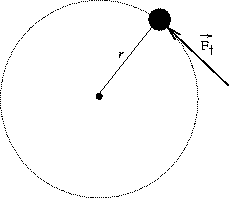Using Newton's second law to relate Ft to the tangential acceleration at = r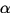, whereis the angular acceleration:

Ft = mat = mr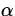and the fact that the torque about the center of rotation due to Ft is:= Ftr , we get: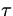= mr 2.

For a rotating rigid body made up of a collection of masses m1,m2.... the total torque about the axis of rotation is: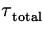=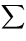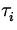= (miri2). (9)
The second line above uses the fact that the angular acceleration of all points in a rigid body is the same, so that it can be taken outside the summation.

Definition: Moment of Inertia of a rigid body

The moment of inertia, I , of a rigid body gives a measure of the amount of resistance a body has to changing its state of rotational motion. Mathematically,

 I =miri2. (10)

Note: The units of moment of inertia are kgm 2.

This allows us to rewrite Equation 8.9 as:= I(11)

which is the rotational analogue of Newton's second law.

Note:

• The complete set of dynamical equations needed to describe the motion of a rigid body consists of the torque equation given above, plus Newton's Second Law applied to the center of mass of the object: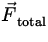= mwhereis the acceleration of the center of mass.
• The moment of inertia, like torque must be defined about a particular axis. It is different for different choices of axes.
• Extended objects can again be considered as a very large collection of much smaller masses glued together to which the definition of moment of inertia given above can be applied.
• Examples of Moments of Inertia of Extended Objects:
uniform hoop: I = mr 2
cylindrical shell I = mr 2
long thin rod (about middle) I =mL 2
long thin rod (about one end) I =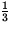mL 2
solid cylinder I =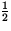mr 2
solid sphere I =mr 2
• The moment of inertia depends on how the mass is distributed about the axis. For a given total mass, the moment of inertia is greater if more mass is farther from the axis than if the same mass is distributed closer to the axis.Next: Rotational Kinetic Energy Up: Rotational Equilibrium and Dynamics Previous: Center of Gravity